# Thermal Resistance to Heat Flow

## Thermal Resistance to Heat Flow:

The Thermal Resistance to Heat Flow between two points in a medium (e.g. insulation) is equal to temperature difference between these points divided by the heat flowing between them in a unit time i e.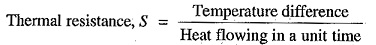In SI units, heat flowing in a unit time is measured in watts.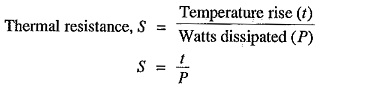Clearly, the SI unit of thermal resistance is °C per watt. This is also called thermal ohm.

Like electrical resistance, thermal resistance is directly proportional to length l in the direction of transmission of heat and inversely proportional to the cross-section area a at right angles to that direction.where k is the constant of proportionality and is known as thermal resistivity.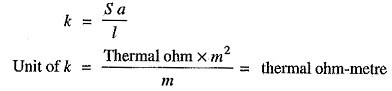### Thermal Resistance of Dielectric of a Single Core Cable:

Let us now find the thermal resistance of the dielectric of a single-core cable.

Let

r = radius of the core in metre

r= inside radius of the sheath in metre

k = thermal resistivity of the insulation (i.e. dielectric)

Consider 1m length of the cable. The thermal resistance of small element of thickness dx at radius x is (See Fig. 11.21)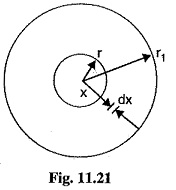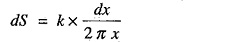Thermal resistance of the dielectric is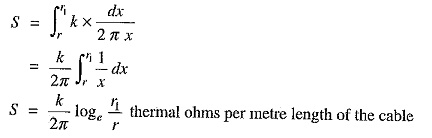The thermal resistance of lead sheath is small and is generally neglected in calculations.

When considering heat dissipation in underground cables, the various thermal resistances providing a heat dissipation path are in series. Therefore, they add up like electrical resistances in series. Consider a cable laid in soil.

Let

I = permissible current per conductor

n = number of conductors

R = electrical resistance per metre length of the conductor at the working temperature

S = total thermal resistance (i.e. sum of thermal resistances of dielectric and soil) per metre length

t = temperature difference (rise) between the conductor and the soil

Neglecting the dielectric and sheath losses, we have,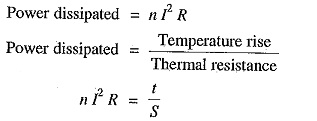Permissible current per conductor is given by;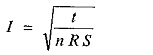It should be noted that when cables are laid in proximity to each other, the permissible current is reduced further on account of mutual heating.